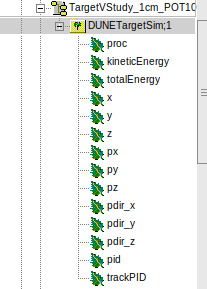# Empty histogram but shows entries

I am trying to read the data from the ROOT file, store that data in the vector and fill the histogram. However, my histogram is showing empty but the entry number is as I expected for that specific particle. Here’s my code:

``````void KEhist()
{
TFile* file1 = new TFile("TargetVStudy_1cm_POT1000.root", "READ");
TTree* tree1 = (TTree*)file1->Get("DUNETargetSim");

Double_t Energy1;
Char_t pid1;
vector<double>nue_1cm;

TH1D* hist1 = new TH1D("nu_e_1cm", "nu_e_1cm Hist", 12000, 0, 120);

Int_t nentries = tree1->GetEntries("kineticEnergy");
//      cout << nentries << endl;

for(Int_t i = 0; i < nentries; i++)
{
tree1->GetEntry(i);
if (strcmp(pid1 , "nu_e") == 0) { nue_1cm.push_back(i); }
}

//      cout << nue_1cm.size() << endl;

for(Int_t j = 0; j < nue_1cm.size(); j++)
{
hist1->Fill(nue_1cm[j]);
}

hist1->Draw();
}

``````Try with:

``````TH1D* hist1 = new TH1D("nu_e_1cm", "nu_e_1cm Hist", 100, 0., 0.); // automatic binning
``````
1 Like